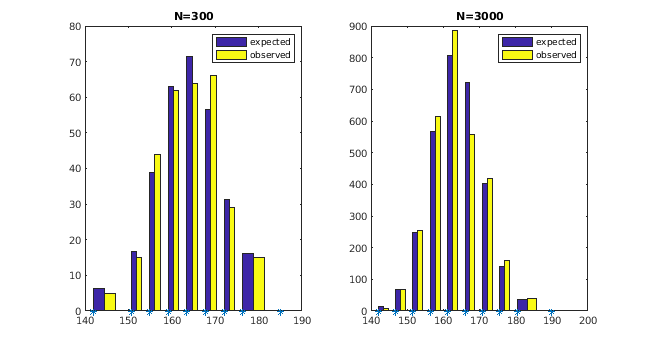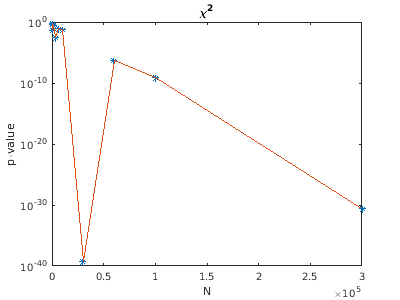### Analysis of body heights

• The problem in a nutshell:
• given two data sets of German female body heights containing N=300 and N=3000 entries
• histogram and Q-Q plot are consistent with normal distribution
• for N=300
•• similar for N=3000
• test for normality with standard χ2 goodness of fit test
• for concreteness: with Matlabs chi2gof
•• results for N = 300
• p-value = 0.5884
• → consistent with normal distribution
• results for N = 3000
• p-value = 6.0958e-10
• → normal distribution is highly unlikely!
• the usual rules of thumb are fulfilled in both cases
• all Oi ≥ 1
• at least 80% of the Oi > 5
• The explanation:
• numerical experiment
• create your own data sets with N data points
• generate values with standard random number generator using normal distribution (μ = 165, σ = 6.9)
• round the values to centimeters
• results of the standard χ2 analysis
• p-value (300) = 0.470
• p-value (3000) = 0.266e-3
• obviously the rounding is the problem
• measured (or rounded) values are from a discrete distribution Ng
• Ng is the "rounded version" of the normal distribution N
• using suitable units → measured values are integer
• Studying behaviour with varying N:
• create rounded values as before
• use chi2gof to compute p-values
• semi-logarithmic plot, using mean values of three runs each
•• findings
• p-value falls drastically (exponentially) with rising N
• but: large fluctuations. e.g.
• N p-values1000 1.67e-01/6.48e-01/4.88e-03 2.73e-01
3000 4.30e-05/7.40e-03/5.36e-04 2.66e-03
• strange behaviour at N=30000
• needs further analysis (cf. below)
• Use Kolmogorow-Smirnow test for comparison:
• reminder
• directly compares empirical and given distribution functions
• strange, but well known test statistics
•